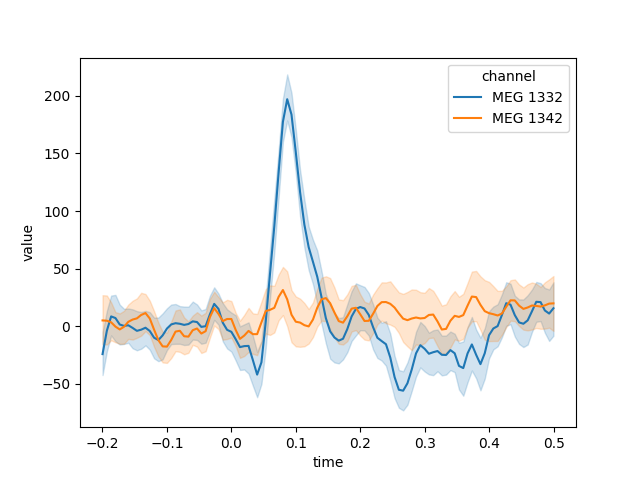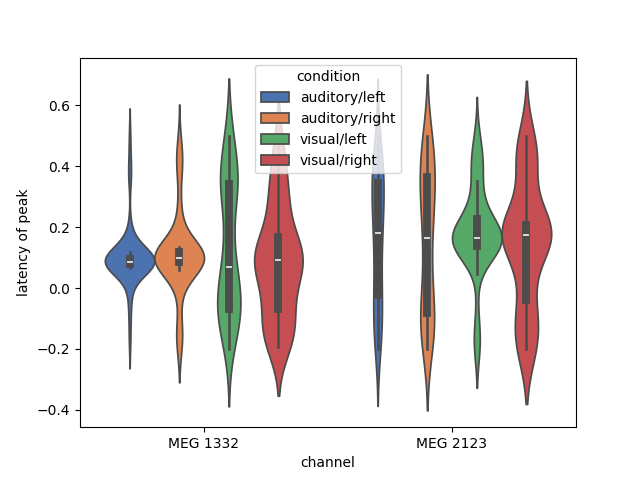# Exporting Epochs to Pandas DataFrames#

This tutorial shows how to export the data in `Epochs` objects to a `Pandas DataFrame`, and applies a typical Pandas split-apply-combine workflow to examine the latencies of the response maxima across epochs and conditions.

We’ll use the Sample dataset, but load a version of the raw file that has already been filtered and downsampled, and has an average reference applied to its EEG channels. As usual we’ll start by importing the modules we need and loading the data:

```import matplotlib.pyplot as plt
import seaborn as sns

import mne

sample_data_folder = mne.datasets.sample.data_path()
sample_data_raw_file = (sample_data_folder / 'MEG' / 'sample' /
'sample_audvis_filt-0-40_raw.fif')
```

Next we’ll load a list of events from file, map them to condition names with an event dictionary, set some signal rejection thresholds (cf. Rejecting Epochs based on channel amplitude), and segment the continuous data into epochs:

```sample_data_events_file = (sample_data_folder / 'MEG' / 'sample' /
'sample_audvis_filt-0-40_raw-eve.fif')

event_dict = {'auditory/left': 1, 'auditory/right': 2, 'visual/left': 3,
'visual/right': 4}

reject_criteria = dict(mag=3000e-15,     # 3000 fT
eeg=100e-6,       # 100 µV
eog=200e-6)       # 200 µV

tmin, tmax = (-0.2, 0.5)  # epoch from 200 ms before event to 500 ms after it
baseline = (None, 0)      # baseline period from start of epoch to time=0

epochs = mne.Epochs(raw, events, event_dict, tmin, tmax, proj=True,
del raw
```
```Not setting metadata
288 matching events found
Setting baseline interval to [-0.19979521315838786, 0.0] sec
Applying baseline correction (mode: mean)
Created an SSP operator (subspace dimension = 4)
4 projection items activated
Rejecting  epoch based on EEG : ['EEG 003']
Rejecting  epoch based on EEG : ['EEG 001', 'EEG 002', 'EEG 003', 'EEG 004', 'EEG 006', 'EEG 007']
Rejecting  epoch based on EEG : ['EEG 001', 'EEG 002', 'EEG 003', 'EEG 007']
Rejecting  epoch based on EEG : ['EEG 001']
Rejecting  epoch based on EEG : ['EEG 003', 'EEG 007']
Rejecting  epoch based on EEG : ['EEG 007']
Rejecting  epoch based on EEG : ['EEG 003', 'EEG 007']
Rejecting  epoch based on EEG : ['EEG 007']
Rejecting  epoch based on MAG : ['MEG 1411', 'MEG 1421', 'MEG 1441']
Rejecting  epoch based on MAG : ['MEG 1711']
Rejecting  epoch based on EEG : ['EEG 001', 'EEG 002', 'EEG 003', 'EEG 007']
Rejecting  epoch based on EEG : ['EEG 007']
Rejecting  epoch based on MAG : ['MEG 1411', 'MEG 1421']
Rejecting  epoch based on MAG : ['MEG 1711']
Rejecting  epoch based on EOG : ['EOG 061']
Rejecting  epoch based on EEG : ['EEG 008']
Rejecting  epoch based on EEG : ['EEG 008']
Rejecting  epoch based on EOG : ['EOG 061']
Rejecting  epoch based on EEG : ['EEG 007', 'EEG 008']
Rejecting  epoch based on EEG : ['EEG 001', 'EEG 002', 'EEG 003', 'EEG 007']
Rejecting  epoch based on EEG : ['EEG 001', 'EEG 003', 'EEG 007']
Rejecting  epoch based on MAG : ['MEG 1411', 'MEG 1421']
Rejecting  epoch based on MAG : ['MEG 1411']
Rejecting  epoch based on EEG : ['EEG 001', 'EEG 002', 'EEG 003', 'EEG 007']
Rejecting  epoch based on EEG : ['EEG 001', 'EEG 002', 'EEG 003', 'EEG 007']
Rejecting  epoch based on EEG : ['EEG 007']
Rejecting  epoch based on EEG : ['EEG 003', 'EEG 007']
Rejecting  epoch based on EEG : ['EEG 003', 'EEG 007']
Rejecting  epoch based on EEG : ['EEG 007']
Rejecting  epoch based on MAG : ['MEG 1331', 'MEG 1421']
Rejecting  epoch based on EEG : ['EEG 007']
Rejecting  epoch based on EOG : ['EOG 061']
Rejecting  epoch based on EEG : ['EEG 001', 'EEG 002', 'EEG 003', 'EEG 007']
Rejecting  epoch based on EEG : ['EEG 001', 'EEG 002', 'EEG 003', 'EEG 007']
Rejecting  epoch based on EEG : ['EEG 001', 'EEG 002', 'EEG 003', 'EEG 007']
```

## Converting an `Epochs` object to a `DataFrame`#

Once we have our `Epochs` object, converting it to a `DataFrame` is simple: just call `epochs.to_data_frame()`. Each channel’s data will be a column of the new `DataFrame`, alongside three additional columns of event name, epoch number, and sample time. Here we’ll just show the first few rows and columns:

```df = epochs.to_data_frame()
df.iloc[:5, :10]
```
time condition epoch MEG 0113 MEG 0112 MEG 0111 MEG 0122 MEG 0123 MEG 0121 MEG 0132
0 -0.199795 visual/left 1 21.320252 11.567619 -73.248584 -10.609895 -18.700540 -68.352746 -17.908667
1 -0.193135 visual/left 1 7.049675 -9.702938 -34.168267 17.480975 -40.182237 -121.225684 2.072452
2 -0.186476 visual/left 1 5.283711 -13.006895 -26.912317 52.130290 -65.157198 -117.842466 6.986073
3 -0.179816 visual/left 1 23.040471 1.643716 -37.835772 48.517952 -65.214631 -82.043144 9.672744
4 -0.173156 visual/left 1 25.688943 7.974112 -85.561683 6.450115 -43.778315 -111.795987 17.277138

### Scaling time and channel values#

By default, time values are converted from seconds to milliseconds and then rounded to the nearest integer; if you don’t want this, you can pass `time_format=None` to keep time as a `float` value in seconds, or convert it to a `Timedelta` value via `time_format='timedelta'`.

Note also that, by default, channel measurement values are scaled so that EEG data are converted to µV, magnetometer data are converted to fT, and gradiometer data are converted to fT/cm. These scalings can be customized through the `scalings` parameter, or suppressed by passing `scalings=dict(eeg=1, mag=1, grad=1)`.

```df = epochs.to_data_frame(time_format=None,
df.iloc[:5, :10]
```
time condition epoch MEG 0113 MEG 0112 MEG 0111 MEG 0122 MEG 0123 MEG 0121 MEG 0132
0 -0.199795 visual/left 1 2.132025e-12 1.156762e-12 -7.324858e-14 -1.060990e-12 -1.870054e-12 -6.835275e-14 -1.790867e-12
1 -0.193135 visual/left 1 7.049675e-13 -9.702938e-13 -3.416827e-14 1.748098e-12 -4.018224e-12 -1.212257e-13 2.072452e-13
2 -0.186476 visual/left 1 5.283711e-13 -1.300690e-12 -2.691232e-14 5.213029e-12 -6.515720e-12 -1.178425e-13 6.986073e-13
3 -0.179816 visual/left 1 2.304047e-12 1.643716e-13 -3.783577e-14 4.851795e-12 -6.521463e-12 -8.204314e-14 9.672744e-13
4 -0.173156 visual/left 1 2.568894e-12 7.974112e-13 -8.556168e-14 6.450115e-13 -4.377831e-12 -1.117960e-13 1.727714e-12

Notice that the time values are no longer integers, and the channel values have changed by several orders of magnitude compared to the earlier DataFrame.

### Setting the `index`#

It is also possible to move one or more of the indicator columns (event name, epoch number, and sample time) into the index, by passing a string or list of strings as the `index` parameter. We’ll also demonstrate here the effect of `time_format='timedelta'`, yielding `Timedelta` values in the “time” column.

```df = epochs.to_data_frame(index=['condition', 'epoch'],
time_format='timedelta')
df.iloc[:5, :10]
```
time MEG 0113 MEG 0112 MEG 0111 MEG 0122 MEG 0123 MEG 0121 MEG 0132 MEG 0133 MEG 0131
condition epoch
visual/left 1 -1 days +23:59:59.800204787 21.320252 11.567619 -73.248584 -10.609895 -18.700540 -68.352746 -17.908667 45.379736 -53.123525
1 -1 days +23:59:59.806864627 7.049675 -9.702938 -34.168267 17.480975 -40.182237 -121.225684 2.072452 27.734826 -95.339465
1 -1 days +23:59:59.813524468 5.283711 -13.006895 -26.912317 52.130290 -65.157198 -117.842466 6.986073 5.996158 -177.627037
1 -1 days +23:59:59.820184308 23.040471 1.643716 -37.835772 48.517952 -65.214631 -82.043144 9.672744 1.748030 -211.024973
1 -1 days +23:59:59.826844149 25.688943 7.974112 -85.561683 6.450115 -43.778315 -111.795987 17.277138 2.123811 -173.917677

### Wide- versus long-format DataFrames#

Another parameter, `long_format`, determines whether each channel’s data is in a separate column of the `DataFrame` (`long_format=False`), or whether the measured values are pivoted into a single `'value'` column with an extra indicator column for the channel name (`long_format=True`). Passing `long_format=True` will also create an extra column `ch_type` indicating the channel type.

```long_df = epochs.to_data_frame(time_format=None, index='condition',
long_format=True)
```
```Converting "epoch" to "category"...
Converting "channel" to "category"...
Converting "ch_type" to "category"...
```
epoch time channel ch_type value
condition
visual/left 1 -0.199795 MEG 0113 grad 21.320252
visual/left 1 -0.199795 MEG 0112 grad 11.567619
visual/left 1 -0.199795 MEG 0111 mag -73.248584
visual/left 1 -0.199795 MEG 0122 grad -10.609895
visual/left 1 -0.199795 MEG 0123 grad -18.700540

Generating the `DataFrame` in long format can be helpful when using other Python modules for subsequent analysis or plotting. For example, here we’ll take data from the “auditory/left” condition, pick a couple MEG channels, and use `seaborn.lineplot()` to automatically plot the mean and confidence band for each channel, with confidence computed across the epochs in the chosen condition:

```plt.figure()
channels = ['MEG 1332', 'MEG 1342']
data = long_df.loc['auditory/left'].query('channel in @channels')
# convert channel column (CategoryDtype → string; for a nicer-looking legend)
data['channel'] = data['channel'].astype(str)
data.reset_index(drop=True, inplace=True)  # speeds things up
sns.lineplot(x='time', y='value', hue='channel', data=data)
```We can also now use all the power of Pandas for grouping and transforming our data. Here, we find the latency of peak activation of 2 gradiometers (one near auditory cortex and one near visual cortex), and plot the distribution of the timing of the peak in each channel as a `violinplot()`:

```plt.figure()
df = epochs.to_data_frame(time_format=None)
peak_latency = (df.filter(regex=r'condition|epoch|MEG 1332|MEG 2123')
.groupby(['condition', 'epoch'])
.aggregate(lambda x: df['time'].iloc[x.idxmax()])
.reset_index()
.melt(id_vars=['condition', 'epoch'],
var_name='channel',
value_name='latency of peak')
)

ax = sns.violinplot(x='channel', y='latency of peak', hue='condition',
data=peak_latency, palette='deep', saturation=1)
```Total running time of the script: ( 0 minutes 13.528 seconds)

Estimated memory usage: 931 MB

Gallery generated by Sphinx-Gallery